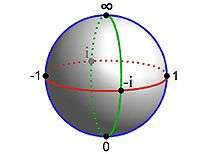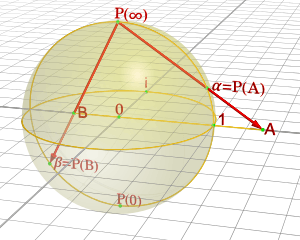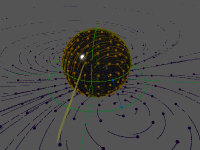# Riemann sphereThe Riemann sphere can be visualized as the complex number plane wrapped around a sphere (by some form of stereographic projection details are given below).

In mathematics, the Riemann sphere, named after Bernhard Riemann, is a model of the extended complex plane, the complex plane plus a point at infinity. This extended plane represents the extended complex numbers, that is, the complex numbers plus a value ∞ for infinity. With the Riemann model, the point "∞" is near to very large numbers, just as the point "0" is near to very small numbers.

The extended complex numbers are useful in complex analysis because they allow for division by zero in some circumstances, in a way that makes expressions such as 1/0 = well-behaved. For example, any rational function on the complex plane can be extended to a continuous function on the Riemann sphere, with the poles of the rational function mapping to infinity. More generally, any meromorphic function can be thought of as a continuous function whose codomain is the Riemann sphere.

In geometry, the Riemann sphere is the prototypical example of a Riemann surface, and is one of the simplest complex manifolds. In projective geometry, the sphere can be thought of as the complex projective line P1(C), the projective space of all complex lines in C2. As with any compact Riemann surface, the sphere may also be viewed as a projective algebraic curve, making it a fundamental example in algebraic geometry. It also finds utility in other disciplines that depend on analysis and geometry, such as quantum mechanics and other branches of physics.

## Extended complex numbers

The extended complex numbers consist of the complex numbers C together with ∞. The set of extended complex numbers may be written as C ∪ {∞}, and is often denoted by adding some decoration to the letter C, such asGeometrically, the set of extended complex numbers is referred to as the Riemann sphere (or extended complex plane).

### Arithmetic operations

Addition of complex numbers may be extended by defining, for z  C,for any complex number z, and multiplication may be defined byfor all nonzero complex numbers z, with ∞ × ∞ = ∞. Note that ∞ + ∞, ∞  ∞ and 0 × ∞ are left undefined. Unlike the complex numbers, the extended complex numbers do not form a field, since ∞ does not have a multiplicative inverse. Nonetheless, it is customary to define division on C  {∞} byfor all nonzero complex numbers z, with /0 = ∞ and 0/ = 0. The quotients 0/0 and / are left undefined.

### Rational functions

Any rational function f(z) = g(z)/h(z) (in other words, f(z) is the ratio of polynomial functions g(z) and h(z) of z with complex coefficients, such that g(z) and h(z) have no common factor) can be extended to a continuous function on the Riemann sphere. Specifically, if z0 is a complex number such that the denominator h(z0) is zero but the numerator g(z0) is nonzero, then f(z0) can be defined as ∞. Moreover, f(∞) can be defined as the limit of f(z) as z → ∞, which may be finite or infinite.

The set of complex rational functions that are not everywhere zero — whose mathematical symbol is C(z) — form all possible holomorphic functions from the Riemann sphere to itself, when it is viewed as a Riemann surface, except for the constant function taking the value ∞ everywhere. The functions of C(z) form an algebraic field, known as the field of rational functions on the sphere.

For example, given the functionwe may define f(5) = ∞, since the denominator is zero at z = 5, and f(∞) = 3 since f(z) → 3 as z → ∞. Using these definitions, f becomes a continuous function from the Riemann sphere to itself.

## As a complex manifold

As a one-dimensional complex manifold, the Riemann sphere can be described by two charts, both with domain equal to the complex number plane C. Let ζ be a complex number in one copy of C, and let ξ be a complex number in another copy of C. Identify each nonzero complex number ζ of the first C with the nonzero complex number 1/ξ of the second C. Then the mapis called the transition map between the two copies of C — the so-called charts — glueing them together. Since the transition maps are holomorphic, they define a complex manifold, called the Riemann sphere. As a complex manifold of 1 complex dimension (i.e., 2 real dimensions), this is also called a Riemann surface.

Intuitively, the transition maps indicate how to glue two planes together to form the Riemann sphere. The planes are glued in an "inside-out" manner, so that they overlap almost everywhere, with each plane contributing just one point (its origin) missing from the other plane. In other words, (almost) every point in the Riemann sphere has both a ζ value and a ξ value, and the two values are related by ζ = 1/ξ. The point where ξ = 0 should then have ζ-value "1/0"; in this sense, the origin of the ξ-chart plays the role of "∞" in the ζ-chart. Symmetrically, the origin of the ζ-chart plays the role of ∞ in the ξ-chart.

Topologically, the resulting space is the one-point compactification of a plane into the sphere. However, the Riemann sphere is not merely a topological sphere. It is a sphere with a well-defined complex structure, so that around every point on the sphere there is a neighborhood that can be biholomorphically identified with C.

On the other hand, the uniformization theorem, a central result in the classification of Riemann surfaces, states that the only simply-connected one-dimensional complex manifolds are the complex plane, the hyperbolic plane, and the Riemann sphere. Of these, the Riemann sphere is the only one that is a closed surface (a compact surface without boundary). Hence the two-dimensional sphere admits a unique complex structure turning it into a one-dimensional complex manifold.

## As the complex projective line

The Riemann sphere can also be defined as the complex projective line. This is the subset of C2 consisting of all pairs (α, β) of complex numbers, not both zero, modulo the equivalence relationfor all nonzero complex numbers λ. The complex plane C, with coordinate ζ, can be mapped into the complex projective line byAnother copy of C with coordinate ξ can be mapped in byThese two complex charts cover the projective line. For nonzero ξ and ζ the following identificationsdemonstrate that the transition maps are ζ = 1/ξ and ξ = 1/ζ, as above.

This treatment of the Riemann sphere connects most readily to projective geometry. For example, any line (or smooth conic) in the complex projective plane is biholomorphic to the complex projective line. It is also convenient for studying the sphere's automorphisms, later in this article.

## As a sphereStereographic projection of a complex number A onto a point α of the Riemann sphere

The Riemann sphere can be visualized as the unit sphere x2 + y2 + z2 = 1 in the three-dimensional real space R3. To this end, consider the stereographic projection from the unit sphere minus the point (0, 0, 1) onto the plane z = 0, which we identify with the complex plane by ζ = x + iy. In Cartesian coordinates (x, y, z) and spherical coordinates (θ, φ) on the sphere (with θ the zenith and φ the azimuth), the projection isSimilarly, stereographic projection from (0, 0, −1) onto the plane z = 0, identified with another copy of the complex plane by ξ = xiy, is writtenIn order to cover the unit sphere, one needs the two stereographic projections: the first will cover the whole sphere except the point (0, 0, 1) and the second except the point (0, 0, −1). Hence, one needs two complex planes, one for each projection, which can be intuitively seen as glued back-to-back at z = 0. Note that the two complex planes are identified differently with the plane z = 0. An orientation-reversal is necessary to maintain consistent orientation on the sphere, and in particular complex conjugation causes the transition maps to be holomorphic.

The transition maps between ζ-coordinates and ξ-coordinates are obtained by composing one projection with the inverse of the other. They turn out to be ζ = 1/ξ and ξ = 1/ζ, as described above. Thus the unit sphere is diffeomorphic to the Riemann sphere.

Under this diffeomorphism, the unit circle in the ζ-chart, the unit circle in the ξ-chart, and the equator of the unit sphere are all identified. The unit disk |ζ| < 1 is identified with the southern hemisphere z < 0, while the unit disk |ξ| < 1 is identified with the northern hemisphere z > 0.

## Metric

A Riemann surface does not come equipped with any particular Riemannian metric. The Riemann surface's conformal structure does, however, determine a class of metrics: all those whose subordinate conformal structure is the given one. In more detail: The complex structure of the Riemann surface does uniquely determine a metric up to conformal equivalence. (Two metrics are said to be conformally equivalent if they differ by multiplication by a positive smooth function.) Conversely, any metric on an oriented surface uniquely determines a complex structure, which depends on the metric only up to conformal equivalence. Complex structures on an oriented surface are therefore in one-to-one correspondence with conformal classes of metrics on that surface.

Within a given conformal class, one can use conformal symmetry to find a representative metric with convenient properties. In particular, there is always a complete metric with constant curvature in any given conformal class.

In the case of the Riemann sphere, the Gauss–Bonnet theorem implies that a constant-curvature metric must have positive curvature K. It follows that the metric must be isometric to the sphere of radius 1/K in R3 via stereographic projection. In the ζ-chart on the Riemann sphere, the metric with K = 1 is given byIn real coordinates ζ = u + iv, the formula isUp to a constant factor, this metric agrees with the standard Fubini–Study metric on complex projective space (of which the Riemann sphere is an example).

Up to scaling, this is the only metric on the sphere whose group of orientation-preserving isometries is 3-dimensional (and none is more than 3-dimensional); that group is called SO(3). In this sense, this is by far the most symmetric metric on the sphere. (The group of all isometries, known as O(3), is also 3-dimensional, but unlike SO(3) is not a connected space.)

Conversely, let S denote the sphere (as an abstract smooth or topological manifold). By the uniformization theorem there exists a unique complex structure on S, up to conformal equivalence. It follows that any metric on S is conformally equivalent to the round metric. All such metrics determine the same conformal geometry. The round metric is therefore not intrinsic to the Riemann sphere, since "roundness" is not an invariant of conformal geometry. The Riemann sphere is only a conformal manifold, not a Riemannian manifold. However, if one needs to do Riemannian geometry on the Riemann sphere, the round metric is a natural choice (with any fixed radius, though radius = 1 is the simplest and most common choice). That is because only a round metric on the Riemann sphere has its isometry group be a 3-dimensional group. (Namely, the group known as SO(3), a continuous ("Lie") group that is topologically the 3-dimensional projective space P3.)

## AutomorphismsA Möbius transformation acting on the sphere, and on the plane by stereographic projection

The study of any mathematical object is aided by an understanding of its group of automorphisms, meaning the maps from the object to itself that preserve the essential structure of the object. In the case of the Riemann sphere, an automorphism is an invertible biholomorphic map from the Riemann sphere to itself. It turns out that the only such maps are the Möbius transformations. These are functions of the formwhere a, b, c, and d are complex numbers such that adbc ≠ 0. Examples of Möbius transformations include dilations, rotations, translations, and complex inversion. In fact, any Möbius transformation can be written as a composition of these.

The Möbius transformations are profitably viewed as transformations on the complex projective line. In projective coordinates, the transformation f can be writtenThus the Möbius transformations can be described as 2 × 2 complex matrices with nonzero determinant; two matrices yield the same Möbius transformation if and only if they differ by a nonzero factor. Thus the Möbius transformations exactly correspond to the projective linear transformations PGL(2, C).

If one endows the Riemann sphere with the Fubini–Study metric, then not all Möbius transformations are isometries; for example, the dilations and translations are not. The isometries form a proper subgroup of PGL(2, C), namely PSU(2). This subgroup is isomorphic to the rotation group SO(3), which is the group of symmetries of the unit sphere in R3 (which, when restricted to the sphere, become the isometries of the sphere).

## Applications

In complex analysis, a meromorphic function on the complex plane (or on any Riemann surface, for that matter) is a ratio f/g of two holomorphic functions f and g. As a map to the complex numbers, it is undefined wherever g is zero. However, it induces a holomorphic map (f, g) to the complex projective line that is well-defined even where g = 0. This construction is helpful in the study of holomorphic and meromorphic functions. For example, on a compact Riemann surface there are no non-constant holomorphic maps to the complex numbers, but holomorphic maps to the complex projective line are abundant.

The Riemann sphere has many uses in physics. In quantum mechanics, points on the complex projective line are natural values for photon polarization states, spin states of massive particles of spin 1/2, and 2-state particles in general (see also Quantum bit). The Riemann sphere has been suggested as a relativistic model for the celestial sphere. In string theory, the worldsheets of strings are Riemann surfaces, and the Riemann sphere, being the simplest Riemann surface, plays a significant role. It is also important in twistor theory.Wikimedia Commons has media related to Riemann sphere.